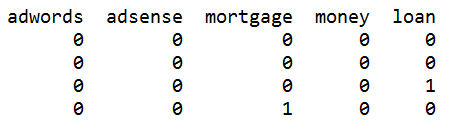# Converting Categorical Text Variable into Binary Variables

Sometimes we might need convert categorical feature into multiple binary features. Such situation emerged while I was implementing decision tree with independent categorical variable using python sklearn.tree for the post Building Decision Trees in Python – Handling Categorical Data and it turned out that a text independent variable is not supported.

One of solution would be binary encoding, also called one-hot-encoding when we might code [‘red’,’green’,’blue’] with 3 columns, one for each category, having 1 when the category match and 0 otherwise. 

Here we implement the python code that makes such binary encoding. The script looks at text data column and add numerical columns with values 0 or 1 to the original data. If category word exists in the column then it will be 1 in the column for this category, otherwise 0.

The list of categories is initialized in the beginning of the script. Additionally we initialize data source file, number of column with text data, and number of first empty column on right side. The script will add columns on right side starting from first empty column.

The next step in the script is to navigate through each row and do binary conversion and update data.

Below is some example of added binary columns to data input .Below is full source code.

``````
# -*- coding: utf-8 -*-

import pandas as pd

total_rows = len(data.index)

y_text_column_index=7
y_column_index=16

for index, w in enumerate(words):
data[w] = 0
col_index=data.columns.get_loc(w)

for x in range (total_rows):
if w in data.iloc[x,y_text_column_index] :
data.iloc[x,y_column_index+index]=1
else :
data.iloc[x,y_column_index+index]=0

print (data)
``````